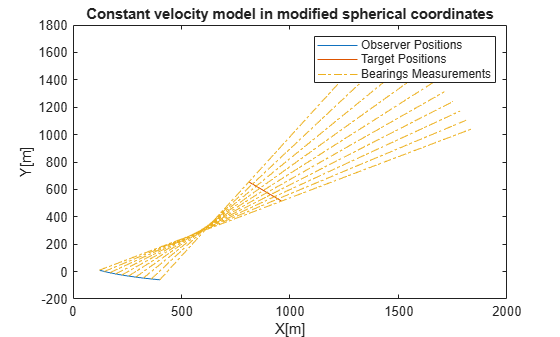# constvelmsc

Constant velocity (CV) motion model in MSC frame

Since R2018b

## Syntax

``state = constvelmsc(state,vNoise)``
``state = constvelmsc(state,vNoise,dt)``
``state = constvelmsc(state,vNoise,dt,u)``

## Description

````state = constvelmsc(state,vNoise)` calculates the state at the next time-step based on current state and target acceleration noise, `vNoise`, in the scenario. The function assumes a time interval, `dt`, of one second, and zero observer acceleration in all dimensions. ```

example

````state = constvelmsc(state,vNoise,dt)` specifies the time interval, `dt`. The function assumes zero observer acceleration in all dimensions.```

example

````state = constvelmsc(state,vNoise,dt,u)` specifies the observer input, `u`, during the time interval, `dt`.```

## Examples

collapse all

Define a state vector for a 3-D MSC state.

```mscState = [0.1;0.01;0.1;0.01;0.001;1]; dt = 0.1;```

Predict the state with zero observer acceleration.

`mscState = constvelmsc(mscState,zeros(3,1),dt)`
```mscState = 6×1 0.1009 0.0083 0.1009 0.0083 0.0009 0.9091 ```

Predict the state with [5;3;1] observer acceleration in scenario.

`mscState = constvelmsc(mscState,zeros(3,1),dt,[5;3;1])`
```mscState = 6×1 0.1017 0.0067 0.1017 0.0069 0.0008 0.8329 ```

Predict the state with observer maneuver and unit standard deviation random noise in target acceleration. Let observer acceleration in the time interval be $\left[\mathrm{sin}\left(\mathit{t}\right)\text{\hspace{0.17em}}\mathrm{cos}\left(\mathit{t}\right)\right]$.

```velManeuver = [1 - cos(dt);sin(dt);0]; posManeuver = [-sin(dt);cos(dt) - 1;0]; u = zeros(6,1); u(1:2:end) = posManeuver; u(2:2:end) = velManeuver; mscState = constvelmsc(mscState,randn(3,1),dt,u)```
```mscState = 6×1 0.1023 0.0058 0.1023 0.0057 0.0008 0.7689 ```

Define a state vector for a motion model in 2-D. The time interval is 2 seconds.

```mscState = [0.5;0.02;1/1000;-10/1000]; dt = 2; ```

As modified spherical coordinates (MSC) state is relative, let the observer state be defined by a constant acceleration model in 2-D.

`observerState = [100;10;0.5;20;-5;0.1];`

Pre-allocate memory. `rPlot` is the range for plotting bearing measurements.

```observerPositions = zeros(2,10); targetPositions = zeros(2,10); azimuthMeasurement = zeros(1,10); bearingHistory = zeros(2,30); rPlot = 2000;```

Use a loop to predict the state multiple times. Use `constvelmsc` to create a trajectory with constant velocity target and measure the angles using the measurement function, `cvmeasmsc`.

```for i = 1:10 obsAcceleration = observerState(3:3:end); % Use zeros(2,1) as process noise to get true predictions mscState = constvelmsc(mscState,zeros(2,1),dt,obsAcceleration); % Update observer state using constant acceleration model observerState = constacc(observerState,dt); observerPositions(:,i) = observerState(1:3:end); % Update bearing history with current measurement. az = cvmeasmsc(mscState); bearingHistory(:,3*i-2) = observerState(1:3:end); bearingHistory(:,3*i-1) = observerState(1:3:end) + [rPlot*cosd(az);rPlot*sind(az)]; bearingHistory(:,3*i) = [NaN;NaN]; % Use the 'rectangular' frame to get relative positions of the % target using cvmeasmsc function. relativePosition = cvmeasmsc(mscState,'rectangular'); relativePosition2D = relativePosition(1:2); targetPositions(:,i) = relativePosition2D + observerPositions(:,i); end```
```plot(observerPositions(1,:),observerPositions(2,:)); hold on; plot(targetPositions(1,:),targetPositions(2,:)); plot(bearingHistory(1,:),bearingHistory(2,:),'-.'); title('Constant velocity model in modified spherical coordinates');xlabel('X[m]'); ylabel('Y[m]') legend('Observer Positions', 'Target Positions', 'Bearings Measurements'); hold off;```## Input Arguments

collapse all

State that is defined relative to an observer in modified spherical coordinates, specified as a vector or a 2-D matrix. For example, if there is a constant velocity target state, xT, and a constant velocity observer state, xO, then the `state` is defined as xT - xO transformed in modified spherical coordinates.

The two-dimensional version of modified spherical coordinates (MSC) is also referred to as the modified polar coordinates (MPC). In the case of:

• 2-D space –– State is equal to [az azRate 1/r vr/r]

• 3-D space –– State is equal to [az omega el elRate 1/r vr/r]

If specified as a matrix, states must be concatenated along columns, where each column represents a state following the convention specified above.

The variables used in the convention are:

• az –– Azimuth angle (rad)

• el –– Elevation angle (rad)

• azRate –– Azimuth rate (rad/s)

• elRate –– Elevation rate (rad/s)

• omega –– azRate × cos(el) (rad/s)

• 1/r –– 1/range (1/m)

• vr/r –– range-rate/range or inverse time-to-go (1/s)

Data Types: `single` | `double`

Target acceleration noise in the scenario, specified as a vector of 2 or 3 elements or a matrix with dimensions corresponding to `state`. That is, if the dimensions of the `state` matrix is 6-by-10, then the acceptable dimensions for `vNoise` is 3-by-10. If the dimensions of the `state` matrix is 4-by-10, then the acceptable dimensions for `vNoise` is 2-by-10. For more details, see Orientation, Position, and Coordinate Convention.

Data Types: `double`

Time difference between current state and the time at which the state is to be calculated, specified as a real finite numeric scalar.

Data Types: `single` | `double`

Observer input, specified as a vector. The observer input can have the following impact on state-prediction based on its dimensions:

• When the number of elements in `u` equals the number of elements in `state`, the input `u` is assumed to be the maneuver performed by the observer during the time interval, `dt`. A maneuver is defined as motion of the observer higher than first order (or constant velocity).

• When the number of elements in `u` equals half the number of elements in `state`, the input `u` is assumed to be constant acceleration of the observer, specified in the scenario frame during the time interval, `dt`.

Data Types: `double`

## Output Arguments

collapse all

State at the next time step, returned as a vector and a matrix of two or three dimensions. The state at the next time step is calculated based on the current state and the target acceleration noise, `vNoise`.

Data Types: `double`

## Algorithms

The function provides a constant velocity transition function in modified spherical coordinates (MSC) using a non-additive noise structure. The MSC frame assumes a single observer and the state is defined relative to it.

## Version History

Introduced in R2018b